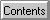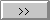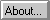# Path Loss

The inverse-square law for electromagnetic radiation implies a free space path loss proportional to the square of the distance.
The loss is given by the following equation:
PathLoss(dB) = -32.45 + 20 * Log(Frequency) + 20 * Log(Distance)
where Frequency is in MHz and Distance is in km.

Converted by Winhelp to Web